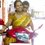# The banana problem

There is a SQUARE field and 44 BANANA trees are planted across the square field. Find the peremeter of that square field, if the distance between 2 BANANA tree is 2 metre.Note by Jeet Dubey
6 years, 8 months ago

This discussion board is a place to discuss our Daily Challenges and the math and science related to those challenges. Explanations are more than just a solution — they should explain the steps and thinking strategies that you used to obtain the solution. Comments should further the discussion of math and science.

When posting on Brilliant:

• Use the emojis to react to an explanation, whether you're congratulating a job well done , or just really confused .
• Ask specific questions about the challenge or the steps in somebody's explanation. Well-posed questions can add a lot to the discussion, but posting "I don't understand!" doesn't help anyone.
• Try to contribute something new to the discussion, whether it is an extension, generalization or other idea related to the challenge.

MarkdownAppears as
*italics* or _italics_ italics
**bold** or __bold__ bold
- bulleted- list
• bulleted
• list
1. numbered2. list
1. numbered
2. list
Note: you must add a full line of space before and after lists for them to show up correctly
paragraph 1paragraph 2

paragraph 1

paragraph 2

[example link](https://brilliant.org)example link
> This is a quote
This is a quote
    # I indented these lines
# 4 spaces, and now they show
# up as a code block.

print "hello world"
# I indented these lines
# 4 spaces, and now they show
# up as a code block.

print "hello world"
MathAppears as
Remember to wrap math in $$ ... $$ or $ ... $ to ensure proper formatting.
2 \times 3 $2 \times 3$
2^{34} $2^{34}$
a_{i-1} $a_{i-1}$
\frac{2}{3} $\frac{2}{3}$
\sqrt{2} $\sqrt{2}$
\sum_{i=1}^3 $\sum_{i=1}^3$
\sin \theta $\sin \theta$
\boxed{123} $\boxed{123}$

Sort by:

88

- 4 years, 5 months ago

4 Banana trees are planted ONE at each corner of a square. and 10 trees are planted at each side between two corners of a side so Perimeter of the square field=4+(10*4)=44 meter

- 6 years, 7 months ago

352 m is right i suppose considering question is right ! 44 banana across the field (assuming the line of 44 bananas parallel to one of the side) having 2 metre between each tree so length of one side comes to 88m . Since perimeter is asked we have to take addition four time because it is square, so 88*4=352.

- 6 years, 7 months ago

Perimeter is 88 m. 12 banana trees per side, 22 metre per side.

- 6 years, 8 months ago

Why are there 12 banana trees per side?

Staff - 6 years, 8 months ago

I thought the 44 trees are in the perimeter...12 per side with 4 in commons in the corners. This is my only way to interpret the problem so that it's logical...

- 6 years, 8 months ago

That's certainly a valid interpretation, given the ambiguity in the question. Thanks for explaining!

Staff - 6 years, 8 months ago

No problem :)

- 6 years, 8 months ago

44 is not a square number so the field can't be square

- 6 years, 8 months ago

I agree that this question doesn't make much sense, and that 144 (likely a typo) would be a much better interpretation.

However, you're making the assumption that the banana trees must be planted parallel to the sides of the field, which is not necessary. Hence, an interpretation of this problem (which likely isn't the one originally intended) could be to find the minimum area of a field that contains exactly 44 banana trees.

Staff - 6 years, 8 months ago

If Jeet D intended to type 144,then the answer would be the same as Luca B's answer.Otherwise it should be $6 \times 2m \times 4=48m$ because 49 is the smallest square number larger than 44 and $\sqrt{49}=7$

- 6 years, 8 months ago

Not quite. This is actually much more interesting. You are still making the same assumption that the banana trees must be planted parallel to the sides of the field.

For example, if we only wanted 2 trees, then your approach will require a square field of side length 2, which has 4 banana trees on the corners. Whereas, if you take the 'diamond', you can see that a square field of side length $\sqrt{2}$ is sufficient, with 2 banana trees on the opposite corners. Can you find an argument to show that this is the minimum length possible?

What happens in the case for 3 banana trees? Can we also do it with a square field of side length $\sqrt{2}$? If not, what is the minimum length?

What happens in the case for 4 banana trees? Is the minimum length 2? Can we not do any better? Why, or why not?

What happens in the case for 44 banana trees?

Staff - 6 years, 8 months ago

Double interpretation with same result LOL

- 6 years, 8 months ago